# Confluent hypergeometric equation

(diff) ← Older revision | Latest revision (diff) | Newer revision → (diff)

degenerate hypergeometric equation

The second-order ordinary linear differential equation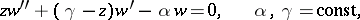(1)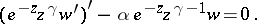In general, the variables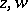and the parameters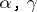may assume complex values. The Whittaker equation is a reduced form of equation (1). Equation (1) is closely connected with the hypergeometric equation. The confluent hypergeometric equation can be regarded as an equation obtained from the Riemann differential equation as a result of the merging of two singular points. The pointis a regular singular point for equation (1), while the pointis a strong singular point (cf. Singular point). E.E. Kummer  was the first to undertake a systematic study of the solutions of equation (1).

The solutions of equation (1) are expressed through the confluent hypergeometric function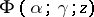. Ifis not an integer, the general solution of equation (1) may be written in the form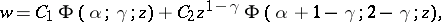(2)

where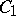and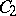are arbitrary constants. This representation is valid in the complex-plane with the slit. Ifis an integer, the general solution has a more complicated form (may contain logarithmic terms). Functions other than those in (2) (e.g. Whittaker functions , ) can also be selected as a fundamental system of solutions of equation (1). Solutions of (1) can also be represented by contour integrals in the complex-plane.

Many second-order ordinary linear differential equations (e.g. the Bessel equation) can be reduced to equation (1) by a transformation of the unknown function and of the independent variable . In particular, equations of the type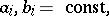can be integrated using the confluent hypergeometric function.

How to Cite This Entry:
Confluent hypergeometric equation. Encyclopedia of Mathematics. URL: http://encyclopediaofmath.org/index.php?title=Confluent_hypergeometric_equation&oldid=13279
This article was adapted from an original article by N.Kh. Rozov (originator), which appeared in Encyclopedia of Mathematics - ISBN 1402006098. See original article